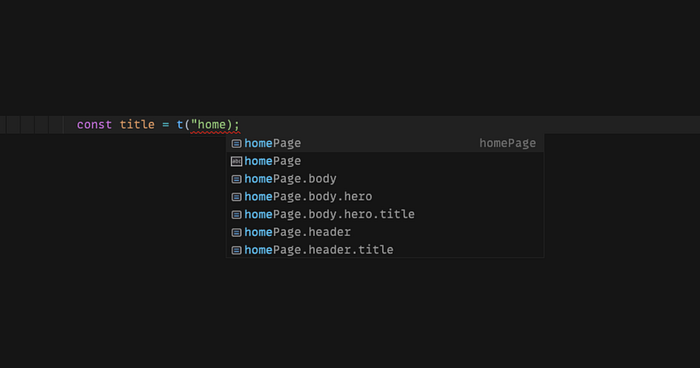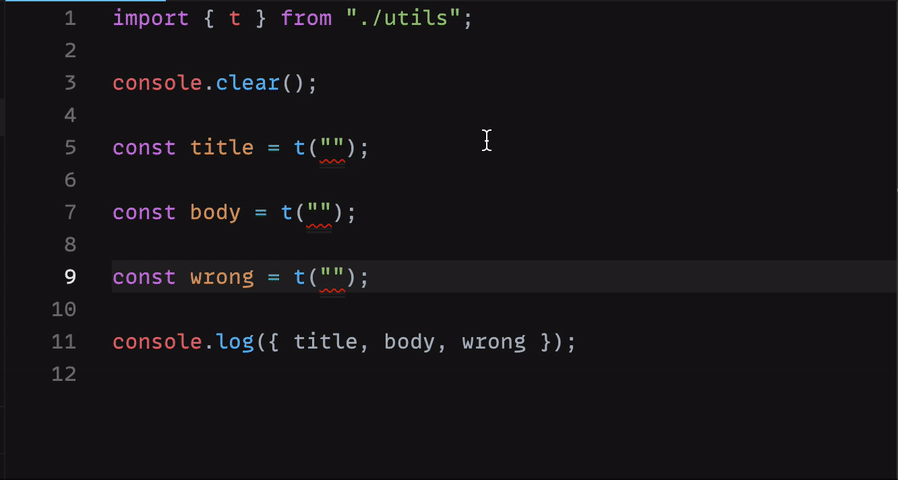# Advanced TypeScript: Type-Level Nested Object Paths

## A Real-world use case of Conditional Types, Type Inference, Template Literal Types, and Recursion.### ❓ The Problem

With Object Path, I mean a dot-case string that indicates the path to a value inside an object, for example:

``````const object = {
a: {
// <-- 'a'
b: "My path is 'a.b'", // <-- 'a.b'
c: "My path is 'a.c'", // <-- 'a.c'
},
d: "My path is just 'd'", // <-- 'd'
};
``````

The path to `My path is ‘a.b’`is `a.b`, the set of all the possible paths of this object is: `'a', 'a.b', 'a.c' and ‘d’`. I often needed a way to know all the possible paths of an object at type-level, for instance, the following example helps to understand a real-world scenario where knowing all these possible paths at type-level can help us to avoid useless conditions or possible errors: How do I know if `_homepage.header.title_` path exists? And even if it exists, how can I be sure that it refers to a string? Of course, I could simply put an if statement, but I wanted something smarter, automatic, and type-checked! I didn’t find anything ready that could solve this problem in a proper way, so I decided to do it by myself and I’ll show you how!

### ✅ Result

In the next chapter, I’ll give you a detailed explanation of the solution I came up with, but first of all, I want to show you the result! — if you’re not interested in the explanation, you can stop here, copy & paste the snippet and you’re good to go!The Types used under the hood are: You didn’t expect it to be that complicated, did you?Me neither, to be honest — But there is a reason why it is like this! Check out the next chapter if you want to know why.

### 📝 Solution Explained

##### NestedPaths

Let’s start from the type `NestedPaths` — I have to say that it could have been made in an easier way, for example like this: That’s way shorter and cleaner, but… there is a caveat in this approach: Both solutions use `[Conditional Types](https://www.typescriptlang.org/docs/handbook/2/conditional-types.html)`, `[Template Literal Types](https://www.typescriptlang.org/docs/handbook/2/template-literal-types.html)`, and `[Recursive Type Reference](https://www.typescriptlang.org/play#example/recursive-type-references)`— There is a little but crucial difference though, this simpler version is not Tail Recursive. In a few words, a function (or, in this case, a type) is tail-recursive if it ends by returning the value of the recursive call;
In this simpler version, in fact, we are not calling the recursion directly, but instead, we call it inside a template literal type inside a union type:

``````K | `\${K}.\${NestedPaths<T[K]>}`;
``````

Why it’s important that the type is Tail Recursive? Because Typescript puts a limit on the number of times a type can recursively call itself, and if it’s not Tail-recursive, this limit is pretty small! How small? It used to be 9, now it’s something like 20 or 50, I don’t remember the exact number but it’s not a lot; If you know it, please drop a comment and I’ll update the article! If the type is Tail Recursive instead, this limit is way higher! (in the official typescript documentation they just say it’s “more generous”). Now that everything it’s clear, let’s take a look at the Tail Recursive version. Union and Join are utility types that avoid unwanted unions or joins between strings and undefined, and make the type more readable:

``````Union<"a", "b">; // --> 'a' | 'b'
Union<undefined, "b">; // --> 'b'
Union<"a", undefined>; // --> 'a'Join<'a', 'b'>        // --> 'a.b'
Join<undefined, "b">; // --> 'b'
Join<"a", undefined>; // --> 'a'
``````

Knowing this, I’ll keep them out of the next snippet, so we can focus better on the NestedPaths type: Let’s see how it works with an example:

``````type Paths = NestedPaths<{
a: {
// <-- a
b: {
// <-- a.b
c: string; // <-- a.b.c
};
d: string; // <-- a.d
};
}>;
``````

We are expecting that the possible paths of this object are:

``````"a" | "a.b" | "a.b.c" | "a.d";
``````

1st round After the first cycle of recursion, this is what the type would look like:

``````type Paths = {
a: NestedPaths<
{
b: { c: string };
d: string;
},
undefined,
"a"
>;
}["a"];
``````

This is because `keyof T` in this case, is just `a` — and for the key `a` we have that:

``````T['a'] = { b: { c: string }, d: string }
// Which means that
T['a'] extends GenericObject ? yes
``````

We are in the first branch of the condition, so:

``````// Prev = undefined
// Path = undefinedNestedPaths<T['a'], Union<Prev, Path>, Join<Path, 'a'>>// Is equal to:
NestedPaths<T["a"], Union<undefined, undefined>, Join<undefined, "a">>; // Which is just:
NestedPaths<T["a"], undefined, "a">; // Prev = undefined, Path = 'a'
``````

2nd round In the second cycle of the recursion we get:

``````type Paths = {
a: {
b: NestedPaths<{ c: string }, "a", "a.b">;
d: "a" | "a.d";
}["b" | "d"];
}["a"];
``````

In this cycle, `keyof T` is `b | d`.
For the key `b` under the sub-object `a` we can repeat the same process we did previously (we are in the first branch of the condition: `T['b'] extends GenericObject`);
For the key`d` instead, the condition `T['d'] extends GenericObject` is false, so we have:

``````// Prev = undefined
// Path = 'a'd: Union<Union<Prev, Path>, Join<Path, 'd'>>;d: Union<Union<undefined, 'a'>, Join<'a', 'd'>>;d: Union<'a', 'a.d'> --> 'a' | 'a.d'
``````

3rd round Now it starts to be interesting… We have basically everything we need:

``````type Paths = {
a: {
b: { c: "a" | "a.b" | "a.b.c" }["c"];
d: "a" | "a.d";
}["b" | "d"];
}["a"];
``````

The key `c` under `b` is resolved in this way:

``````c: T['c'] extends GenericObject ? nope!c: Union<Union<Prev, Path>, Join<Path, 'c'>>// Where:
// Prev = 'a'
// Path = 'a.b'c: Union<Union<'a', 'a.b'>, Join<'a.b', 'c'>>
c: Union<'a' | 'a.b', 'a.b.c'>
c: 'a' | 'a.b' | 'a.b.c'
``````

Finally The recursion is finished, we just have to write the type in a different way:

``````type Paths = {
a: {
b: { c: "a" | "a.b" | "a.b.c" }["c"];
d: "a" | "a.d";
}["b" | "d"];
}["a"]; // Is equal to:
type Paths = {
a: {
b: "a" | "a.b" | "a.b.c";
d: "a" | "a.d";
}["b" | "d"];
}["a"]; // Which is also equal to:
type Paths = {
a: "a" | "a.b" | "a.b.c" | "a.d";
}["a"]; // 🎉 Which is just what we expected 🎉:
type Paths = "a" | "a.b" | "a.b.c" | "a.d";
``````

With the type NestedPaths, we can get all the paths of any object! …But this was just the first problem I wanted to solve!

##### TypeFromPath

Other than getting all the paths of an object, in fact, I wanted to find a way to also get the type that any of these paths refer to, this is how I did it: Let’s see also in this case, with a concrete example, how this type works:

``````type Result = TypeFromPath<
{
a: {
b: {
c: { foo: "bar" }; // <-- 'a.b.c'
};
d: string;
};
// We are asking for the type referred by the path 'a.b.c'
},
"a.b.c"
>;
``````

We expect that `type Result` will be equal to `{ foo: 'bar' }` . 1st round This is what we get in the first cycle of recursion:

``````type Result = {
"a.b.c": _TypeFromPath_<
{
b: {
c: { foo: "bar" };
};
d: string;
},
"b.c"
>;
}["a.b.c"];
``````

Let’s see what happened:

``````// The generic type Path is equal to 'a.b.c' so
// Path = 'a.b.c'{
[K in Path]: ???
}[Path]// Is just
{
[K in 'a.b.c']: ???
}['a.b.c']
``````

What do we have instead of `???`?
Knowing that `K` is equal to ‘a.b.c’, we have:

``````// T = { a: { ... }, d: string }
// Does _K_ extends keyof _T_?
// No it doesn't, the keys of T are '_a_' and '_d_' and _K_ is '_a.b.c_'
// so we are in the second branch of the condition{
[K in 'a.b.c']: K extends `\${infer P}.\${infer S}` ? TypeFromPath<T[P], S> : never
}['a.b.c']
``````

What does K extends `\${infer P}.\${infer S}` means?
We are asking Typescript if `a.b.c` extends a string that is something like `{Prefix}.{Suffix}`;
In our case it’s true, in fact, K is`a.b.c`, and we can see `a.b.c` as `\${'a'}.\${'b.c'}`. So, in the end, we got:

``````{
['a.b.c']: TypeFromPath<T['a'], 'b.c'>;
}['a.b.c']
``````

2nd round In the second cycle of the recursion we get:

``````type Result = {
"a.b.c": {
"b.c": _TypeFromPath_<
{
c: { foo: "bar" };
},
"c"
>;
}["b.c"];
}["a.b.c"];
``````

In this cycle we have mostly the same thing as before, so:

``````// Path = 'b.c'
// T = { b: { c: { foo: 'bar' } } }; {
[K in 'b.c']: TypeFromPath<T['b'], 'c'>;
}['b.c']// All together:
{
['a.b.c']: {
['b.c']: TypeFromPath<T['b'], 'c'>;
}['b.c']
}['a.b.c']
``````

3rd round Here we finally have something different:

``````type Result = {
"a.b.c": {
"b.c": {
c: { foo: "bar" };
}["c"];
}["b.c"];
}["a.b.c"];
``````

This is how we got it:

``````// Path = 'c'
// T = { c: { foo: 'bar' } };{
[K in 'c']: K extends keyof T ? yes!
}['b.c']{
[K in 'c']: T['c'] --> { foo: 'bar' }
}// All together:
{
['a.b.c']: {
['b.c']: {
['c']: { foo: 'bar' }
}['c]
}['b.c']
}['a.b.c']
``````

4th round We have now everything we need to get the type referred by ‘a.b.c’:

``````type Result = {
"a.b.c": {
"b.c": { foo: "bar" };
}["b.c"];
}["a.b.c"]; // Which is equal to
type Result = {
"a.b.c": { foo: "bar" };
}["a.b.c"]; // And finally
type Result = { foo: "bar" };
``````

Just as we expected, `type Result = { foo: 'bar' };`!

### 💡 Use Cases

I think that these types can be useful in different scenarios, for example, Translations and Redux selectors:

##### Translations

Usually, we store translations in objects like the following one:

``````const translations = {
homePage: {
title: 'Hello!',
...,
},
profilePage: {
settings: 'Settings',
...,
},
},
...,
}
``````

And then we usually use this object through a hook, like the following`useTranslations`, that returns the `t` function — this function takes a path as an argument and returns the corresponding translation:

``````const HomePageTitle = () => {
const { t } = useTranslation();

return <h1>{t("homePage.title")}</h1>;
};
``````

This is how most of the i18n libraries works, for example `i18next` (`react-i18next`) or `react-intl` . We can wrap `useTranslation`into a custom hook and use the types NestedPaths and TypeFromPath to correctly type-check the path passed to the t-function: That’s it, by using this new hook we will always be sure that the path passed to the t-function is a valid path for the translations object, and also that the returned type is a string:

``````const _HomePageTitle_ = () => {
const { t } = useTranslation();

// Looks good
return <h1>{t('homePage.title')}</h1>;
}const _WrongHomePageTitle_ = () => {
const { t } = useTranslation();

// We will have an error saying that homePage.wrongPath
// is not a valid path
return <h1>{t('homePage.wrongPath')}</h1>;
}const _AnotherWrongHomePageTitle_ = () => {
const { t } = useTranslation();

// We will have an error saying that t('homePage')
// is not a valid React child (_because is an object_)
return <h1>{t('homePage')}</h1>;
}
``````
##### Redux selectors

Another great use case could be combining these types with the hook`useSelector` of `react-redux`, for example: